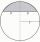# Airplane

Aviator sees part of the earth's surface with an area of 200,000 square kilometers. How high he flies?

Result

h =  4.995 km

#### Solution:Leave us a comment of example and its solution (i.e. if it is still somewhat unclear...):Be the first to comment!#### To solve this example are needed these knowledge from mathematics:

Pythagorean theorem is the base for the right triangle calculator. See also our trigonometric triangle calculator.

## Next similar examples:

1. PilotHow high is the airplane's pilot to see 0.001 of Earth's surface?
2. Spherical capPlace a part of the sphere on a 4.6 cm cylinder so that the surface of this section is 20 cm2. Determine the radius r of the sphere from which the spherical cap was cut.
3. Spherical capWhat is the surface area of a spherical cap, the base diameter 20 m, height 2 m.
4. Spherical cap 4What is the surface area of a spherical cap, the base diameter 20 m, height 2.5 m? Calculate using formula.
5. CalculateCalculate the length of a side of the equilateral triangle with an area of 50cm2.
6. Triangle ABCIn a triangle ABC with the side BC of length 2 cm The middle point of AB. Points L and M split AC side into three equal lines. KLM is isosceles triangle with a right angle at the point K. Determine the lengths of the sides AB, AC triangle ABC.
7. Euclid2In right triangle ABC with right angle at C is given side a=27 and height v=12. Calculate the perimeter of the triangle.
8. Euklid4Legs of a right triangle have dimensions 244 m and 246 m. Calculate the length of the hypotenuse and the height of this right triangle.
9. Equilateral triangleThe equilateral triangle has a 23 cm long side. Calculate its content area.
10. Isosceles trapeziumCalculate the area of an isosceles trapezium ABCD if a = 10cm, b = 5cm, c = 4cm.
11. Theorem proveWe want to prove the sentence: If the natural number n is divisible by six, then n is divisible by three. From what assumption we started?
12. Isosceles IVIn an isosceles triangle ABC is |AC| = |BC| = 13 and |AB| = 10. Calculate the radius of the inscribed (r) and described (R) circle.
13. Circle annulusThere are 2 concentric circles in the figure. Chord of larger circle 10 cm long is tangent to the smaller circle. What are does annulus have?
14. ABS CNCalculate the absolute value of complex number -15-29i.
15. Vector 7Given vector OA(12,16) and vector OB(4,1). Find vector AB and vector |A|.
16. MedianIn triangle ABC is given side a=10 cm and median ta= 13 cm and angle gamma 90°. Calculate length of the median tb.
17. Isosceles triangleThe leg of the isosceles triangle is 5 dm, its height is 20 cm longer than the base. Calculate base length z.